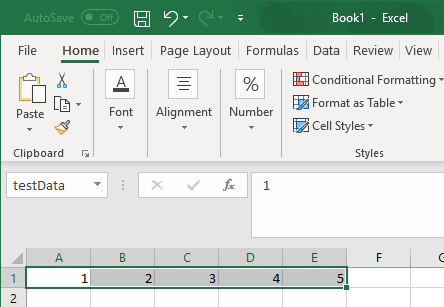# MLPutMatrix

Create or overwrite MATLAB matrix with data from Microsoft Excel worksheet

## Syntax

``= MLPutMatrix(var_name, mdat)``
``MLPutMatrix var_name, mdat``
``out = MLPutMatrix(var_name,mdat)``

## Description

example

````= MLPutMatrix(var_name, mdat)` creates or overwrites the matrix `var_name` in the MATLAB® Workspace with the data specified in `mdat`. The function `MLPutMatrix` creates `var_name` if it does not exist. Use this syntax when working directly in a worksheet.```

example

````MLPutMatrix var_name, mdat` creates or overwrites the matrix `var_name` in the MATLAB Workspace with the data specified in `mdat`. Use this syntax in a VBA macro.```
````out = MLPutMatrix(var_name,mdat)` lets you find errors when executing `MLPutMatrix` in a VBA macro. If `MLPutMatrix` fails, then `out` is a string containing an error code. Otherwise, `out` is `0`.```

## Examples

collapse all

Create a matrix in the MATLAB Workspace using the `MLPutMatrix` function in an Excel® worksheet.

Enter the values 1 through 5 in cells `A1` through `E1`.

Define the name `testData` for the range of cells `A1` through `E1`. For instructions on defining names, see Excel Help.

The range name `testData` appears in the Name Box when the range is selected.Execute the `MLPutMatrix` function in cell `A2`. Use `A` as the name of the matrix to create. Specify the range name `testData` as the data to include in the matrix.

`= MLPutMatrix("A", testData)`

After you press Enter, Excel creates the matrix in the MATLAB Workspace. The matrix contains the data included in the `testData` cell range.

Create a matrix in the MATLAB Workspace using the `MLPutMatrix` function in a VBA macro.

Enter the values 1 through 5 in cells `A1` through `E1`.

Define the name `testData` for the range of cells `A1` through `E1`. For instructions on defining names, see Excel Help.

The range name `testData` appears in the Name Box when the range is selected.On the Developer tab in Excel, click in the Code group. The Visual Basic® Editor window opens.

Select Insert > Module to insert a new module. In the Module1 window, enter this VBA code containing a macro named `PutMatrix`.

```Sub PutMatrix() MLPutMatrix "A", testData End Sub```

The `PutMatrix` macro uses the `MLPutMatrix` function to create the matrix `A` in the MATLAB Workspace using the data in the cell range `testData`.

For details about working with modules, see Excel Help.

Select any worksheet cell. Run the macro by clicking button on the VBA toolbar. For details about running macros, see Excel Help.

The `MLPutMatrix` function creates the matrix in the MATLAB Workspace.

## Input Arguments

collapse all

Name of the MATLAB matrix to create or overwrite, specified as a string.

`var_name` in quotes directly specifies the matrix name. `var_name` without quotes specifies a worksheet cell address (or range name) that contains the matrix name.

Example: `"A"`

Location of the data to copy into `var_name`, specified as a string.

`mdat` must be a worksheet cell address or range name. Do not enclose the location in quotes.

Example: `testData`

Example: `A1`

## Tips

• If `var_name` exists, the `MLPutMatrix` function replaces its contents with the contents of `mdat`.

• Empty numeric data cells in `mdat` become numeric zeros in the MATLAB matrix identified by `var_name`.

• If any element of `mdat` contains string data, `mdat` becomes a MATLAB cell array. Empty string elements in `mdat` become `NaN`s in the MATLAB cell array.

• When using `MLPutMatrix` in a subroutine, indicate the source of the worksheet data using the Microsoft® Excel macro `Range`. For example:

```Sub test() MLPutMatrix "a", Range("A1:A3") End Sub ```

If you have a named range in your worksheet, you can specify the name instead of the range. For example:

```Sub test() MLPutMatrix "a", Range("temp") End Sub ```
• To work with VBA code in Excel with Spreadsheet Link™, you must enable Spreadsheet Link as a reference in the Microsoft Visual Basic Editor. For details, see Installation.

Introduced before R2006a